Function Repository Resource:

# HasseDiagram

Construct a Hasse diagram of a poset

Contributed by: Wolfram Staff (original content by Sriram V. Pemmaraju and Steven S. Skiena)
 ResourceFunction["HasseDiagram"][f,s] constructs a Hasse diagram of the partial order set (poset) defined by the binary relation f and set s.

## Details and Options

ResourceFunction["HasseDiagram"][f,s] effectively constructs the transitive reduction graph of the relation graph defined by the binary relation f and set s.
ResourceFunction["HasseDiagram"] accepts the options of Graph.

## Examples

### Basic Examples (3)

The Hasse diagram of the set of all subsets of a three-element set, ordered by inclusion:

 In:=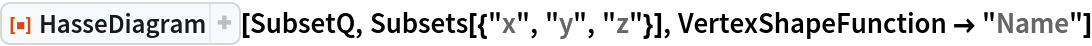Out=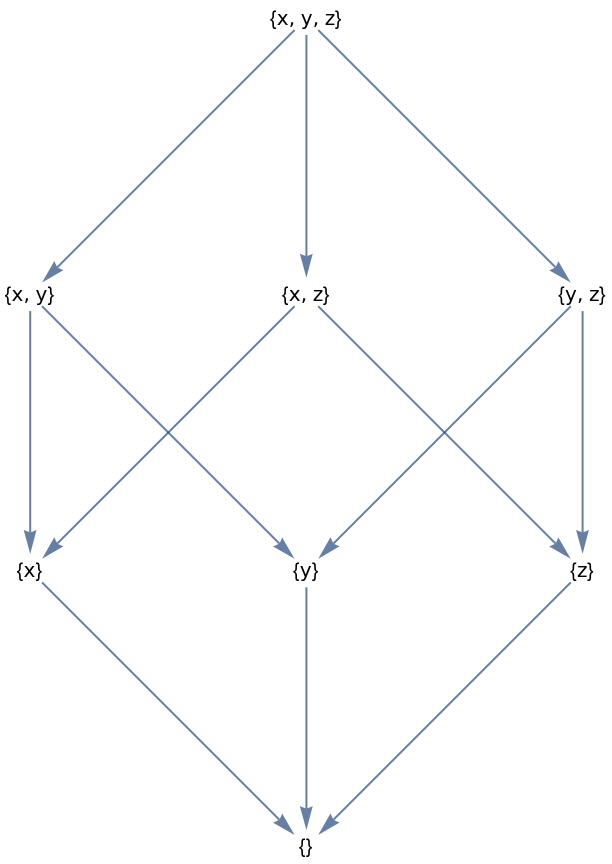Permutations differing by one transposition, ordered by the number of inversions:

 In:=Out=Positive integers ordered by divisibility:

 In:=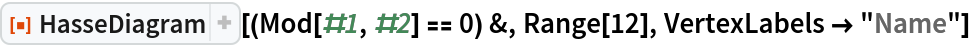Out=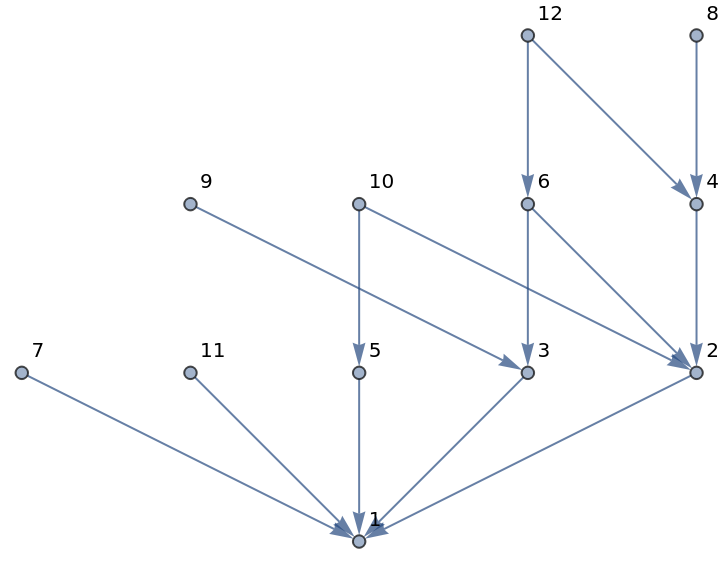### Applications (1)

Display the domination lattice on integer partitions using the resource function DominatingIntegerPartitionQ:

 In:=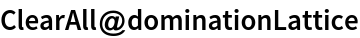In:=In:=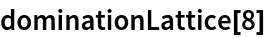Out=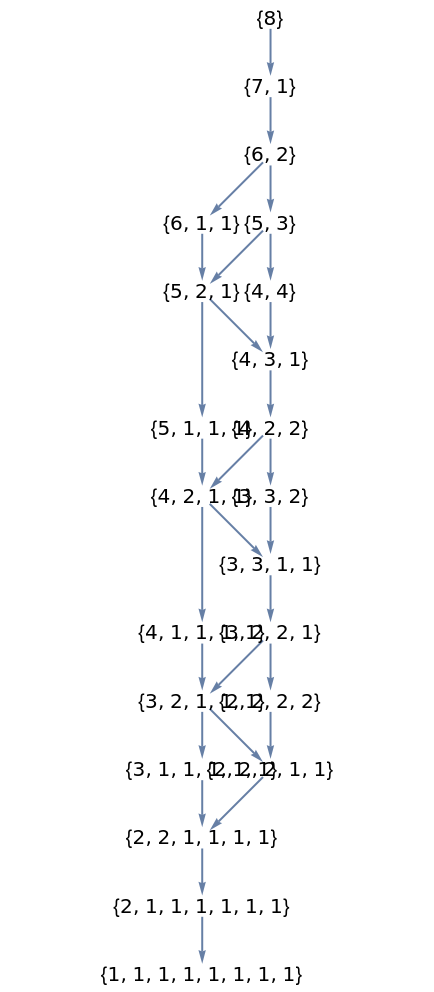## Version History

• 1.0.0 – 28 July 2020